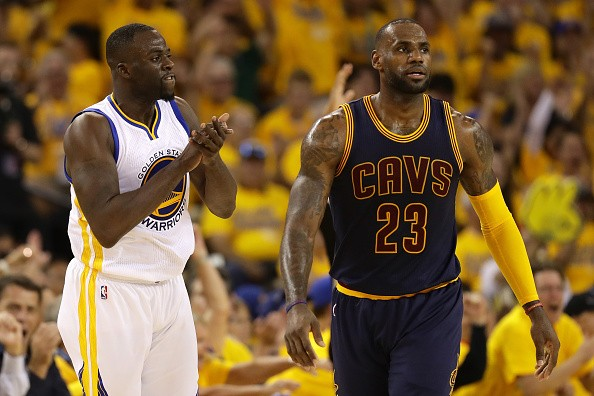# 2017-18 NBA Title Odds##### 2017-18 NBA Title Odds

The 2016-17 NBA Finals hadn’t even started before sportsbooks were starting to take bets on the 2017-18 title. No surprise that the Warriors and Cavs are tops again, and here are opening and current odds:

TeamNovember 15
(5Dimes)
October 17
(5Dimes)
July 7
(5Dimes)
July 1
(5Dimes)
June 28
(Westgate)
June 23
(5Dimes)
June 13
(BetOnline)
May 28
(Westgate)
Warriors-175-187-168-163-220-168-150-160
Cavs+540+515+350+350+300+330+450+250
Celtics+1390+1200+750+900+1500+1400+1600+1500
Rockets+1425+2150+1050+1050+1500+3300+2500+3000
Spurs+1625+1800+1000+1000+1200+1200+1000+1000
Thunder+2500+2150+3000+3000+8000+15000+5000+6000
Wizards+3750+9000+2800+2800+4000+5000+4000+3000
Timberwolves+6600+5000+3500+5000+6000+6600+10000+10000
Bucks+6600+8000+4500+4500+6000+6600+5000+6000
Raptors+6600+15000+5000+5000+8000+6600+5000+6000
Clippers+10000+19000+6600+6600+10000+6600+4000+3000
76ers+15000+19000+10000+10000+8000+25000+10000+10000
Heat+17500+24000+7000+8000+8000+12500+12500+10000
Nuggets+20000+20000+10000+10000+10000+25000+10000+10000
Grizzlies+20000+37000+10000+10000+20000+12500+8000+10000
Hornets+24500+50000+15000+15000+30000+40000+12500+30000
Jazz+25000+25000+3300+3300+10000+15000+5000+10000
Blazers+25000+31500+12500+12500+10000+15000+12500+10000
Pelicans+27500+22000+6000+6000+8000+10000+5000+8000
Pistons+30000+63000+15000+15000+20000+30000+12500+20000
Magic+30000+250000+50000+50000+100000+100000+100000+100000
Pacers+50000+125000+20000+20000+30000+20000+10000+10000
Lakers+100000+30000+10000+10000+10000+12500+10000+10000
Mavericks+100000+50000+15000+15000+20000+25000+12500+20000
Knicks+100000+100000+15000+15000+50000+30000+15000+30000
Nets+100000+250000+50000+50000+100000+100000+500000+100000
Kings+200000+125000+50000+50000+100000+100000+500000+100000
Hawks+200000+200000+20000+20000+20000+30000+15000+20000
Bulls+200000+250000+10000+10000+50000+20000+10000+10000
Suns+999999+125000+50000+50000+50000+100000+50000+50000

For reference, the Warriors opened +150 last year and Cavs opened +250. By the start of last season, the Warriors were -125 and the Cavs +225, similar to the odds we’re seeing now for the upcoming 2017-18 season.

At 5Dimes, you can bet on a Warriors/Cavs finals matchup vs. the field:
Warriors vs. Cavaliers (+175)
Any other matchup (-225)

Check out conference odds as well:

Eastern Conference

Team5Dimes (11/15)5Dimes (10/17)BetOnline (7/6)Westgate (6/24)
Cleveland-140-165-140-250
Boston+310+245+275+450
Washington+1100+2200+1000+1200
Milwaukee+1725+2000+1400+1500
Toronto+1725+3100+1400+2000
Miami+4600+4000+4000+2000
Charlotte+6600+7500+5000+10000
Orlando+7500+25000+3300+40000
Detroit+7500+9000+5000+6000
Indiana+11000+14000+6600+10000
New York+20000+12000+5000+20000
Brooklyn+20000+25000+25000+40000
Atlanta+35000+21000+8000+6000
Chicago +35000+25000+10000+20000

Western Conference

Team5Dimes (11/15)5Dimes (10/17)BetOnline (7/6)Westgate (6/24)
Golden State-245-355-325-500
Houston+780+1100+600+1500
San Antonio+915+1000+600+650
Oklahoma City+1350+1100+2500+4000
Minnesota+3000+2600+2000+3000
Los Angeles Clippers+4750+8000+5000+2000
Denver+6600+8000+6600+5000
Memphis+6600+12500+6600+10000
Utah+7500+9000+4000+5000
Portland+7500+10500+8000+5000
New Orleans+8000+8300+4000+5000
Los Angeles Lakers+20000+9500+5000+5000
Dallas+20000+13500+10000+10000
Sacramento+35000+32500+350000+50000
Phoenix+100000+32500+350000+25000

We’ll continue to update the odds until the season begins and throughout the year. Will the Warriors and Cavs meet for a 4th consecutive NBA Finals? Is it worth betting on anyone else?

##### Dan McGuire

Dan McGuire is the Operations Manager and soccer specialist at Sports Insights. You can find him on Twitter (@ArsenalDMC) and he can be reached directly at dan.mcguire@actionnetwork.com.

• ###### Abdul Aziz Awal
07/29/2017 at 2:34 am

Good